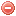# Description

The Numerical risk index was developed by Sol (1990 in order to improve the prediction of fire occurrence and spread (in one overall number) in southern France (cf. Orieux index; Orieux 1974).

The Numerical risk takes air humidity, soil water reserve and wind speed into account (Sol 1990. It requires therefore daily air temperature, dew point temperature, cloud cover, wind speed and potential evapotranspiration (according to Thornthwaite 1948) as input variables.

In contrast to the Orieux index which was only suitable in summer, the Numerical risk allows for fire danger rating also in winter and spring.

# Formula

The Numerical risk $$RN$$ 'is calculated as follows (Sol 1990):

$RN=25-\dfrac{FH\cdot{WRF}\cdot{WF}}{15}+RSF$

where $$FH$$ is false relative humidity, $$WRF$$ the soil water reserve factor, $$WF$$ the wind factor, and $$RSF$$ the rate of spread correction factor.

$$FH$$ is calculated as follows:

$FH=100\cdot{\dfrac{e_{s}(T_{dew})}{e_{s}(T_{soil})}}$

where $$e_{s}(T_{dew})$$ is the saturation vapor pressure [hPa] at the dew point temperature [°C], and $$e_{s}(T_{soil})$$ the saturation vapor pressure [hPa] at the soil (litter) temperature [°C]. The formula of saturation vapor pressure at temperature $$T$$ can be found on the page concerning the vapor pressure deficit. NB: The saturation vapor pressure based on this formula has to be multiplied by 10 in order to be expressed in hPa.

The $$T_{soil}$$ is derived as follows (temperature at the soil or litter temperature) (Camia & Bovio 2000):

$T_{soil}= \begin{cases} 0.874\cdot{T}-0.189\cdot{U}+21.38, & \mbox{for }Cc\leqslant{2} \\ &\\ 1.36\cdot{T}-1.422\cdot{Cc}-0.22\cdot{T_{dew}}+13.42, & \mbox{for }Cc\geqslant{3} \\ \end{cases}$

where $$T$$ is air temperature [°C], $$U$$ wind speed [km/h], $$Cc$$ cloud cover [Okta], and $$T_{dew}$$ dew point temperature [Â°C].

The soil water reserve factor $$WRF$$ is calculated as follows:

$WRF=3+2\cdot{\tanh \bigg(\dfrac{r-50}{25}}\bigg)$

where $$\mathrm{tanh}$$ is the hyperbolic tangent and $$r$$ the soil water reserve [mm] according to the Orieux (1974) definition (cf. Orieux index).

The wind factor $$WF$$ is calculated as follows:

$WF=3+3\cdot\tanh \bigg(\dfrac{45-U}{50}\bigg)$

The rate of spread correction factor $$RSF$$ is determined as follows:

$RSF= \begin{cases} -3, & \mbox{for }ROS\leqslant{600} \\ &\\ 0, & \mbox{for }600 < ROS < 1000 \\ &\\ 2, & \mbox{for }ROS\geqslant{1000} \\ \end{cases}$

where $$ROS$$, the rate of spread [m/h], is calculated as follows:

$ROS=180\cdot e^{ T\cdot{1714}}\cdot\tanh \bigg(\dfrac{100-r}{150}\bigg)\cdot\Bigg\{1+2\cdot\bigg(0.8483+\tanh \bigg(\dfrac{U}{30}-1.25\bigg)\bigg)\Bigg\}$

NB1: In Camia & Bovio (2000), the exponent $$T\cdot1714$$ of the exponential function in the $$ROS$$ equation is written $$T\cdot{0.06}$$

NB2: If the Numerical risk is calculated in winter or spring, the value of the soil water reserve $$r$$ has to be set to 45 [mm].

The Numerical risk could also be calculated on a hourly basis. However the water reserve $$r$$ is only calculated on a daily basis.

According to the conditions for the Orieux index, the computation should started when the water reserve $$r$$ is saturated (150 [mm]), as e.g. after strong rainfall. The value of $$r$$ is then set to 150.

# Index interpretation

The Numerical risk has to be interpreted as follows:

Index values Interpretation
< 10 low fire danger
10 -15 high fire danger
15 -20 very high fire danger

Original publication:
Sol (1990)

Other publications:
Thornthwaite (1948)
Orieux (1974)
Camia & Bovio (2000)

### Bibliography SearchNo results!

No results were found. Are you sure you searched for a tag?

### Symbols

 Variable Description Unit $$T$$ air temperature °C $$T_{dew}$$ dew point temperature °C $$H$$ air humidity % $$P$$ rainfall mm $$U$$ windspeed m/s $$w$$ days since last rain (or rain above threshold) d $$rr$$ days with consecutive rain d $$\Delta t$$ time increment d $$\Delta{e}$$ vapor pressure deficit kPa $$e_s$$ saturation vapor pressure kPa $$e_a$$ actual vapor pressure kPa $$p_{atm}$$ atmospheric pressure kPa $$PET$$ potential evapotranspiration mm/d $$r$$ soil water reserve mm $$r_s$$ surface water reserve mm $$EMC$$ equilibrium moisture content % $$DF$$ drought factor - $$N$$ daylight hours hr $$D$$ weighted 24-hr average moisture condition hr $$\omega$$ sunset hour angle rad $$\delta$$ solar declination rad $$\varphi$$ latitude rad $$Cc$$ cloud cover Okta $$J$$ day of the year (1..365/366) - $$I$$ heat index - $$R_n$$ net radiation MJ⋅m-2⋅d-1 $$R_a$$ daily extraterrestrial radiation MJ⋅m-2⋅d-1 $$R_s$$ solar radiation MJ⋅m-2⋅d-1 $$R_{so}$$ clear-sky solar radiation MJ⋅m-2⋅d-1 $$R_{ns}$$ net shortwave radiation MJ⋅m-2⋅d-1 $$R_{nl}$$ net longwave radiation MJ⋅m-2⋅d-1 $$\lambda$$ latent heat of vaporization MJ/kg $$z$$ elevation m a.s.l. $$d_r$$ inverse relative distance Earth-Sun - $$\alpha$$ albedo or canopy reflection coefficient - $$\Delta$$ slope of the saturation vapor pressure curve kPa/°C $$Cc$$ cloud cover eights $$ROS$$ rate of spread m/h $$RSF$$ rate of spread factor - $$WF$$ wind factor - $$WRF$$ water reserve factor - $$FH$$ false relative humidity - $$FAF$$ fuel availability factor - $$PC$$ phenological coefficient -

 Suffix Description $$-$$ mean / daily value $$_{max}$$ maximum value $$_{min}$$ minimum value $$_{12}$$ value at 12:00 $$_{13}$$ value at 13:00 $$_{15}$$ value at 15:00 $$_{m}$$ montly value $$_{y}$$ yearly value $$_{f/a}$$ value at fuel-atmosphere interface $$_{dur}$$ duration $$_{soil}$$ value at soil level

 Constant Description $$e$$ Euler's number $$\gamma$$ psychrometric constant $$G_{SC}$$ solar constant $$\sigma$$ Stefan-Bolzmann constant